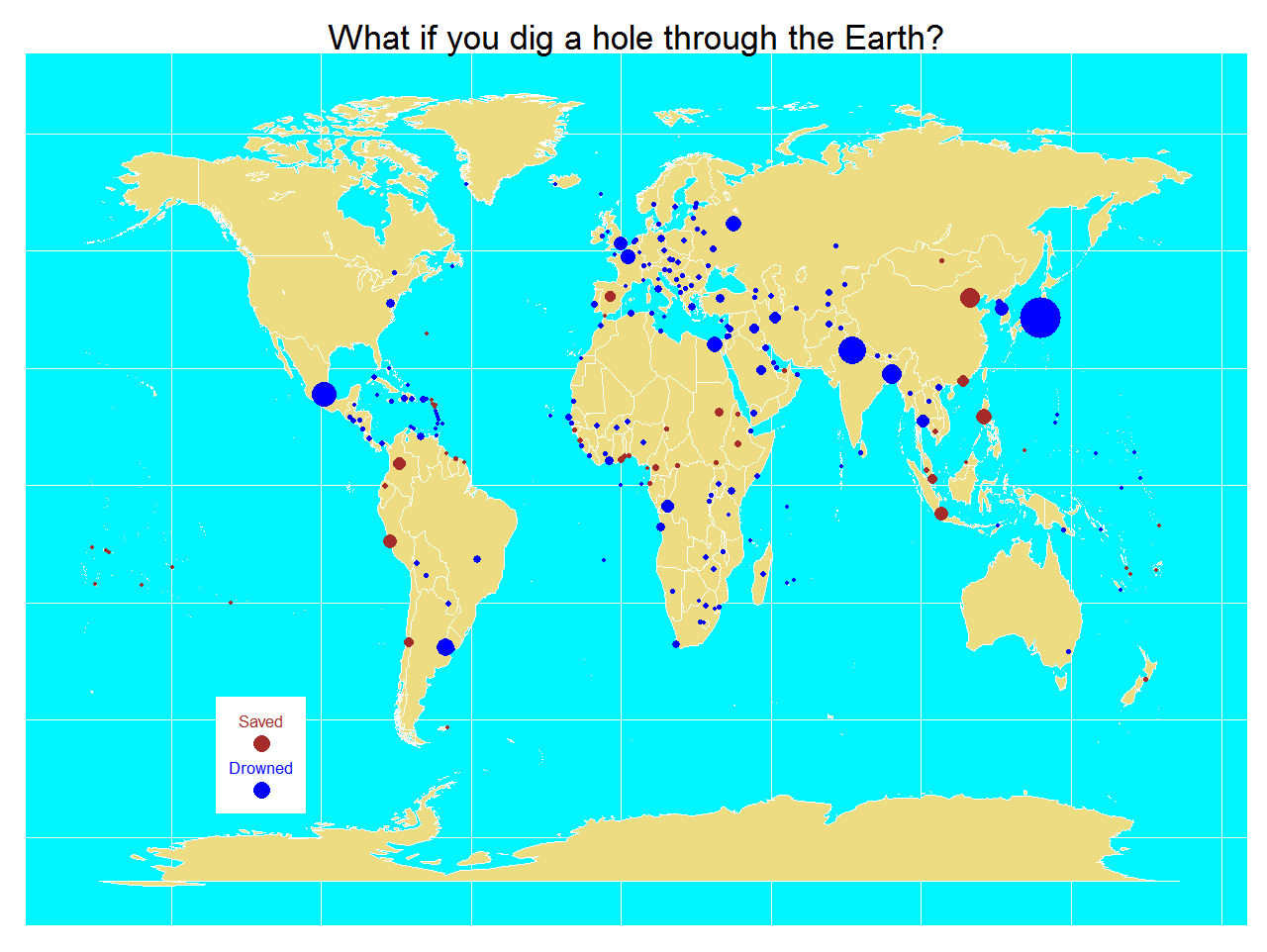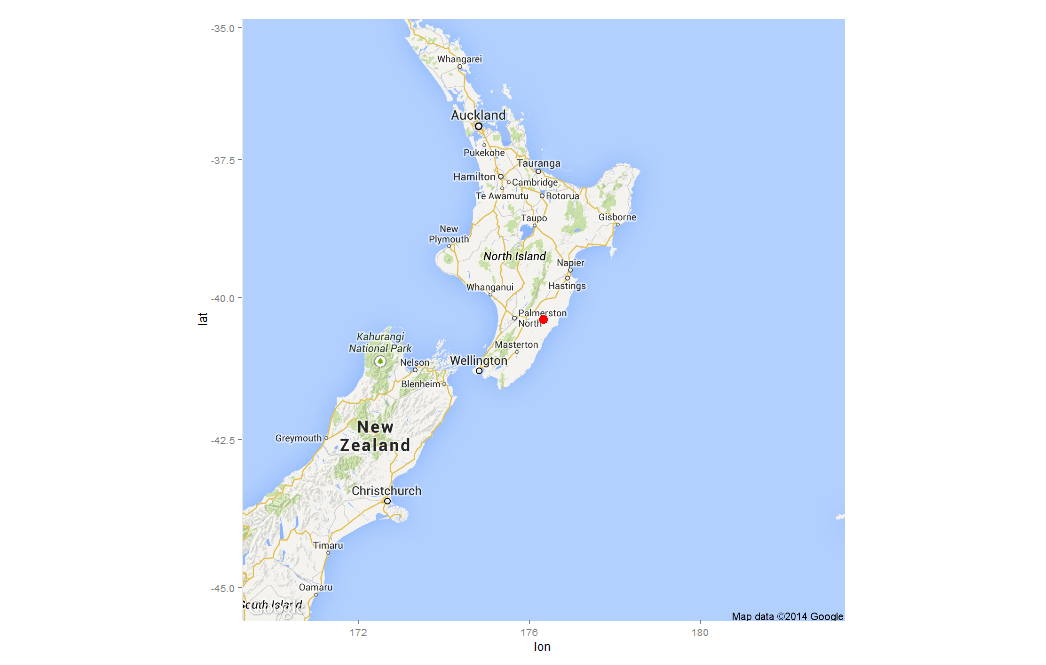# What If You Dig A Hole Through The Earth?

It suddenly struck me that that tiny pea, pretty and blue, was the Earth. I put up my thumb and shut one eye, and my thumb blotted out the planet Earth. I didn’t feel like a giant. I felt very, very small (Neil Armstrong)

Where would you come out if you dig a hole straight downward from where you live through the center of the Earth? Supposing you survive to the extremely high pressure and temperature of the nucleus, would you find water or land at the other side? It maybe sound a ridiculous question (I will not refute that) but in this post I will estimate how many people would die drowned and how many will find land in the antipode of where they live. At least, knowledge does not take up any space.

I found a database of the United Nations with very useful information for my experiment: longitude, latitude and population of all capital cities of the world in 2011(1). I assumed that capital cities are a good sample of where people live. Maybe is not the best one since some very big countries are represented by only a city but is a good way to obtain a quick estimation. On the other hand, capital cities represent approximately 7% of the world population so in this sense is a very good sample.

Process is simple: loading the xls file, calculating the antipode of each point and checking where it is. Google provides information about country where a coordinate belongs. For coordinates on the sea, no information is returned. Once you have this, is easy to calculate proportion of people that will find water. My estimation is around 77% of people will find water on the other side. Taking into account that all people leave from land and approximately 70% of the Earth’s surface is water, this figure seems to be small but since both poles are symmetrical and are uninhabited, the estimation makes sense. Here you have a map with the result of the experimet. Points are capital cities and size is related with population. In blue, capitals with antipode on the sea and in brown, capitals with antipode in land:By the way, I am one of the 23% of lucky people that would find land in the other side. I live in Madrid, Spain:An if some rainy afternoon having little to do I dig a hole through the Earth, I will appear in a place called Weber, in New Zealand:This estimation can be very silly but the physics involved in the experiment are very interesting as you can see here. By the way, there is a film called Total Recall (2012) where the only way to travel between last two cities in the world is using an elevator through the Earth. Here you have the code:

```library(xlsx)
library(ggmap)
library(mapdata)
library(ggplot2)
#The xls file is in http://esa.un.org/unpd/wup/CD-ROM/WUP2011-F13-Capital_Cities.xls
names(CapitalCities) = gsub("\\.", "", names(CapitalCities))
#Obtain symmetric coordinates for each capital
CapitalCities\$LatitudeSym <- -CapitalCities\$Latitude
CapitalCities\$LongitudeSym <- -sign(CapitalCities\$Longitude)*(180-abs(CapitalCities\$Longitude))
CapitalCities\$DigResult <- apply(CapitalCities, 1, function(x) {unlist(revgeocode(c(as.numeric(x),as.numeric(x))))})
CapitalCities\$Drowned <- is.na(CapitalCities\$DigResult)*1
#Percentage of population saved
sum(CapitalCities\$Drowned*CapitalCities\$Populationthousands)/sum(CapitalCities\$Populationthousands)
world <- map_data("world")
opt <- theme(legend.position="none",
axis.ticks=element_blank(),
axis.title=element_blank(),
axis.text =element_blank(),
plot.title = element_text(size = 35),
panel.background = element_rect(fill="turquoise1"))
p <- ggplot()
p <- p + geom_polygon(data=world, aes(x=long, y=lat, group = group),colour="white", fill="lightgoldenrod2" )
p <- p + geom_point(data=CapitalCities, aes(x=Longitude, y=Latitude, color=Drowned, size = Populationthousands)) + scale_size(range = c(2, 20), name="Population (thousands)")
p <- p + labs(title = "What if you dig a hole through the Earth?")
p <- p + scale_colour_gradient(low = "brown", high = "blue")
p <- p + annotate("rect", xmin = -135, xmax = -105, ymin = -70, ymax = -45, fill = "white")
p <- p + annotate("text", label = "Drowned", x = -120, y = -60, size = 6, colour = "blue")
p <- p + annotate("text", label = "Saved", x = -120, y = -50, size = 6, colour = "brown")
p <- p + geom_point(aes(x = -120, y = -65), size=8, colour="blue")
p <- p + geom_point(aes(x = -120, y = -55), size=8, colour = "brown")
p + opt
# Get a map of Spain, centered and signed in Madrid